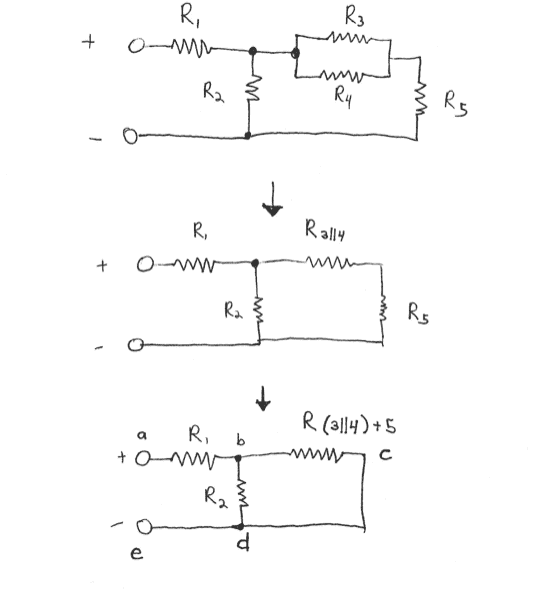# Circuits: Draw Equivalent Resistance

## Homework Statement

Man, I stink at circuits. I have to simplify the 1st circuit in the image below to an equivalent resistance. I am almost there, but I am unsure of how to relate the remaining 3 resistances. In the drawing, a subscript Ri||j denotes the equivalent resistance of the two parallel resistors i and j; similarly, Ri+j is the equivalent resistance of the two series resistors i and j.

Now, I am not sure how to relate $R_1,\,R_{(3||4)+5},\,R_2$ and I think my confusion lies in the fact that I do not know what is going on with the current in this last circuit. Can someone help me out with that?Homework Helper
You have it pretty well finished! Just combine the R(3||4)+5 with the R2 in parallel. That result is in series with R1.

You have it pretty well finished! Just combine the R(3||4)+5 with the R2 in parallel. That result is in series with R1.

OK! Now, this is because after the current leaves R1, it splits among R2 and R(3||4)+5 so we combine them in parallel. Right?

Thanks again Delphi!

Mentor
They combine in parallel because they *are* in parallel! Look at how their leads connect.